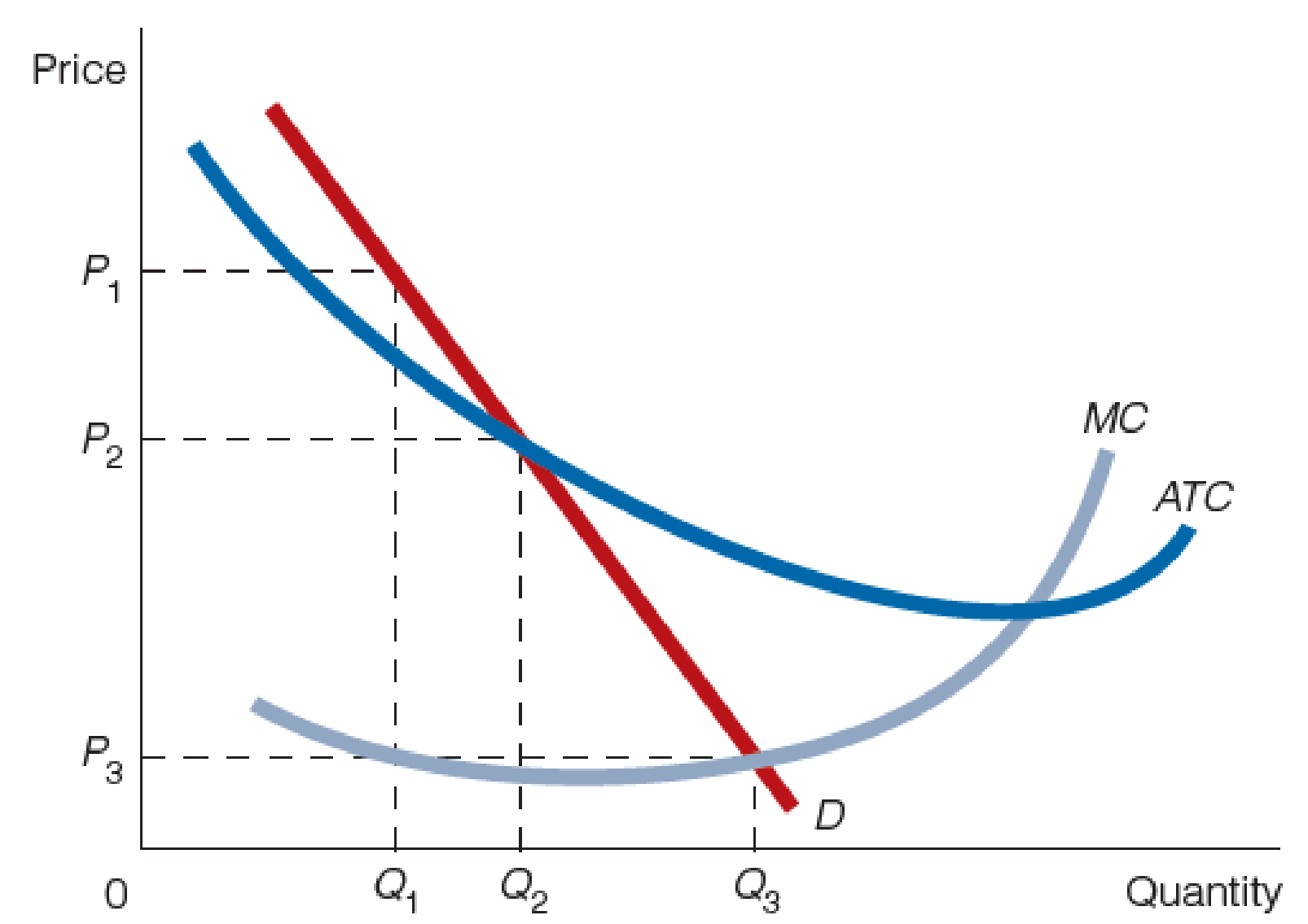Chapter 25, Problem 4WNG

Chapter
Section
Textbook Problem

Use the accompanying figure to answer questions 2-4.4. Which quantity in the figure is consistent with profit regulation? With price regulation? Explain your answers.

To determine

Determine the quantity in the figure that is consistent with profit regulation and price regulation.

Explanation

Figure-1 shows the natural monopoly market as follows:

In Figure-1, the horizontal axis measures the quantity and the vertical axis measures the price.

According to the profit regulation, the government will regulate the profit of the firm by charging the marker price equal to the average total cost (ATC), where the natural monopoly earns zero economic profit...

Still sussing out bartleby?

Check out a sample textbook solution.

See a sample solution

The Solution to Your Study Problems

Bartleby provides explanations to thousands of textbook problems written by our experts, many with advanced degrees!

Get Started